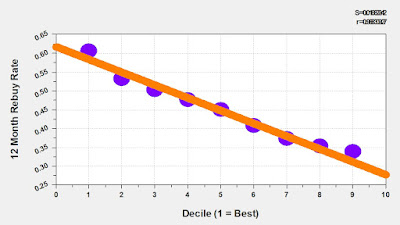## November 13, 2017

### The MineThatData Forecasting Challenge: Solution #1

Here is the three month result (rolling 12 month demand):
• Month 80 = \$81.9 million.
• Month 81 = \$81.3 million.
• Month 82 = \$80.5 million.
Here is their three month forecast:
• Month 80 = \$81.7 million.
• Month 81 = \$81.5 million.
• Month 82 = \$81.3 million.
Here is their "squared error":
• 0.7 Trillion.
Solution #2 is tomorrow - we'll see how this solution compares!